## Example Questions

### Example Question #21 : Algebra

Which of the following is the equation of a line parallel to the line given by the equation: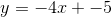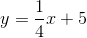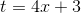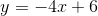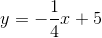Explanation:

Parallel lines have the same slope and different y-intercepts. If their y-intercepts and slopes are the same they are the same line, and therefore not parallel. Thus the only one that fits the description is:### Example Question #22 : Algebra

A line passes through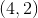and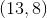. Give the equation, in slope-intercept form, of a parallel line that passes through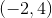.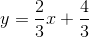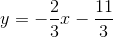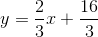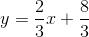Explanation:

The first step in solving for a parallel line is to find the slope of the original line. In this case,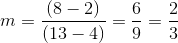.

Our new line has an equal slope (the definition of parallel) and passes through, so substituting into our slope-intercept equation gives us:--->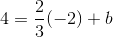--->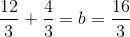.

Thus, the slope intercept equation for our parallel line is.

### Example Question #31 : Algebra

What is the equation of the line passing through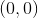and which is parallel to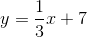?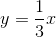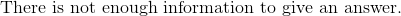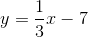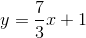Explanation:

Ordinarily, we would use point-slope form,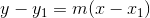, to construct a proper parallel from a point and a slope. However, in this case, we have been given a y-intercept by the problem itself, so sticking with slope-intercept form is easiest.

If the new line passes through, then we know the y-intercept is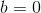. Since slope is equal in parallel lines, and the slope of our comparison line is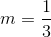, we know the slope of our new line is.

Thus,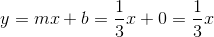.

The correct answer is,.

### Example Question #32 : Algebra

Which of the following equations represents a line that is parallel to the line represented by the equation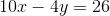?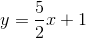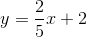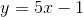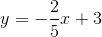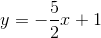Explanation:

Lines are parallel when their slopes are the same.

First, we need to place the given equation in the slope-intercept form.Subtract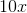from both sides of the equation.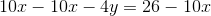Simplify.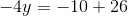Divide both sides of the equation by.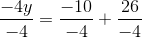Simplify.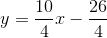Reduce.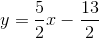Because the given line has the slope of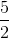, the line parallel to it must also have the same slope.

### Example Question #4 : Coordinate Geometry

If the line through the points (5, –3) and (–2, p) is parallel to the line y = –2x – 3, what is the value of p ?

0

17

4

10

11

11

Explanation:

Since the lines are parallel, the slopes must be the same. Therefore, (p+3) divided by (25) must equal 2. 11 is the only choice that makes that equation true. This can be solved by setting up the equation and solving for p, or by plugging in the other answer choices for p.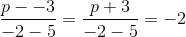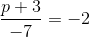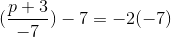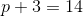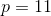### Example Question #1 : How To Find The Slope Of Parallel Lines

What is the slope of a line parallel to the line: -15x + 5y = 30 ?

30

1/3

-15

3

3

Explanation:

First, put the equation in slope-intercept form: y = 3x + 6. From there we can see the slope of this line is 3 and since the slope of any line parallel to another line is the same, the slope will also be 3.

### Example Question #4 : How To Find The Slope Of Parallel Lines

What is the slope of any line parallel to –6x + 5y = 12?

6

12/5

6/5

5/6

12

6/5

Explanation:

This problem requires an understanding of the makeup of an equation of a line.  This problem gives an equation of a line in y = mx + b form, but we will need to algebraically manipulate the equation to determine its slope.  Once we have determined the slope of the line given we can determine the slope of any line parallel to it, becasue parallel lines have identical slopes.  By dividing both sides of the equation by 5, we are able to obtain an equation for this line that is in a more recognizable y = mx + b form. The equation of the line then becomes y = 6/5x + 12/5, we can see that the slope of this line is 6/5.

### Example Question #5 : How To Find The Slope Of Parallel Lines

What is the slope of a line that is parallel to the line 11x + 4y - 2 = 9 – 4x  ?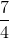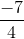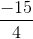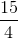Explanation:

We rearrange the line to express it in slope intercept form.

Any line parallel to this original line will have the same slope.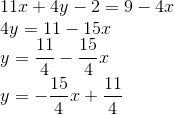### Example Question #1 : How To Find The Slope Of Parallel Lines

In the standard (x, y) coordinate plane, what is the slope of a line parallel to the line with equation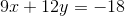?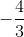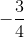Explanation:

Parallel lines will have equal slopes. To solve, we simply need to rearrange the given equation into slope-intercept form to find its slope.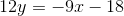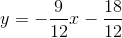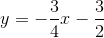The slope of the given line is. Any lines that run parallel to the given line will also have a slope of.

### Example Question #1 : How To Find The Slope Of Parallel Lines

What is the slope of a line that is parallel to the line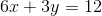?Explanation:

Parallel lines have the same slope. The question requires you to find the slope of the given function. The best way to do this is to put the equation in slope-intercept form (y = mx + b) by solving for y.

First subtract 6x on both sides to get 3y = –6x + 12.

Then divide each term by 3 to get y = –2x + 4.

In the form y = mx + b, m represents the slope. So the coefficient of the x term is the slope, and –2 is the correct answer.